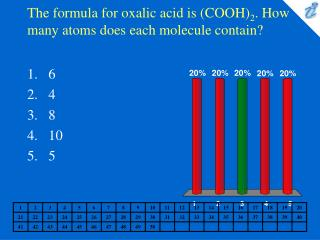DownloadDownload PresentationThe formula for oxalic acid is (COOH) 2 . How many atoms does each molecule contain?

# The formula for oxalic acid is (COOH) 2 . How many atoms does each molecule contain?

Télécharger la présentation## The formula for oxalic acid is (COOH) 2 . How many atoms does each molecule contain?

- - - - - - - - - - - - - - - - - - - - - - - - - - - E N D - - - - - - - - - - - - - - - - - - - - - - - - - - -
##### Presentation Transcript

1. The formula for oxalic acid is (COOH)2. How many atoms does each molecule contain? • 6 • 4 • 8 • 10 • 5

2. What is the molecular formula for acetic acid? • CH3COOH • CH3COCH3 • CH3CH2OH • CH3CH2CO2H • CH3CH2OCH2CH3

3. What is the formula for iron(III) oxide? • FeO3 • FeO2 • Fe3O2 • Fe2O3 • Fe3O

4. Choose the name-formula pair that does not correctly match. • magnesium phosphate Mg3(PO4)2 • ferrous sulfite FeSO3 • silver carbonate AgCO3 • potassium fluoride KF • cupric bromide CuBr2

5. If 6.653 g of calcium combines exactly with 26.53 g of bromine and 12.29 g of zinc combines exactly with 30.04 g of bromine, what is the mass ratio of one atom of calcium to one atom of zinc? • 1.68 • 0.613 • 0.541 • 1.85 • 0.133

6. Calculate the number of atoms in 40.5 g of aluminum. • 900 • 2.5 x 10-24 • 9.0 x 1023 • 6.6 x 1026 • 1.8 x 10-21

7. What is the formula weight of Fe(NH4)2(SO4)2•6H2O? • 392 amu • 384 amu • 412 amu • 376 amu • 436 amu

8. How many atoms of hydrogen are there in 88 g of C6H6? • 3.6 x 1023 • 6.8 x 1022 • 2.6 x 1023 • 4.1 x 1024 • 5.3 x 1023

9. How many hydrogen atoms are present in 7.50 g of (NH4)2Fe(SO4)2•6H2O? • 5.16 x 1022 • 2.30 x 1023 • 1.15 x 1022 • 4.65 x 1023 • 3.35 x 1024

10. Calculate the percent composition of FeSO4. • %Fe = 27.9%, %S = 24.1, %O = 48.0% • %Fe = 16.2% , %S = 27.9%, %O = 55.8% • %Fe = 33.2% , S = 9.5% , %O = 57.2% • %Fe = 36.8%, S = 21.1%, %O = 42.1% • %Fe = 25.1%, %S = 21.6% , %O = 53.2%

11. What would be the empirical formula for a compound containing 33.36% calcium, 26.69% sulfur, and 39.95% oxygen? • CaSO4 • CaSO2 • CaSO • CaSO3 • Ca2S2O5

12. The complete combustion of a hydrocarbon produced 352 mg of CO2 and 216 mg of H2O. What is the simplest formula of this hydrocarbon? • CH • CH • C2H3 • CH3 • C3H2

13. The complete combustion of a hydrocarbon produced 26.4 g of CO2 and 5.40 g of H2O. Another experiment determined the molecular weight of this hydrocarbon to be approximately 52 g/mol. What is its molecular formula? • C2H4 • CH • C4H4 • C2H2 • CH4

14. What mass of lead is present in 5.05 kg of mendipite, PbCl2•2PbO? • 2.89 kg • 4.05 kg • 5.05 kg • 3.68 kg • 4.33 kg

15. What mass of fluorine is contained in 2.00 tons of cryolite that is 42.0% pure Na3A1F6? (No other compounds containing fluorine are present.) • 912 lb • 832 lb • 456 lb • 304 lb • 152 lb